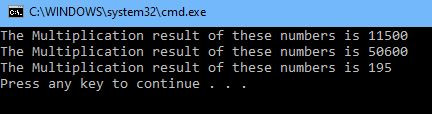Search

# Lesson #12 - Working with Methods

Updated: Dec 13, 2020

A method is a block of code that is used when it is called by a specific request. Methods are very important as they reduce the use of code lines and enhance the clarity, debugging, and efficiency of the program.

There are different types of methods that we can use in our program, for example, a function that only runs a code block without receiving or returning a value or a function that can receive parameters and return a value.

## Creating your first method

A method is declared with a few mandatory parts that will determine how we can access this method and how it can be used in the program.

class Program

{

static void Main(string[] args)

{

MyFirstMethod(); //Call a method

}

private static void MyFirstMethod() //Method

{

//Code to be executed

}

}

Let's review this example by breaking up the method structure:

• Private – The security level of the method is configured as private (We can only access it from the same class).

• Static – This means that the method is part of the program class and not as an object of the program class (I will cover this topic in future posts).

• Void – This means that the method will not need to return any value besides just running its code block.

• MyFirstMethod – This is just the method name that we must specify in order to call the method.

• () – This is where we specify the different parameters that the method is expected to receive prior It can execute its code block.

As you can see, we can pass information to methods by just using it as parameters. Parameters are then used as variables as part of the code execution of the method.

Here are two simple examples that can demonstrate it:

Example 1:

class Program

{

static void Main(string[] args)

{

NumberMultiplication(100,115);//Call Method #1

NumberMultiplication(44,1150); //Call Method #2

NumberMultiplication(13, 15); //Call Method #3

}

private static void NumberMultiplication(int firstNumaber, int secondNumber)

{

Console.WriteLine("The Multiplication result of these numbers is {0}", firstNumaber*secondNumber);

}

}

Result:## Working with Method variables

As I mentioned above methods can be used with a set of parameters sent to them (or not) when we trigger them in the code. it is now important to understand how those parameters are affected before and after the block code is executed.

### Example 1: No impact on the original variable

class Program

{

static void Main(string[] args)

{

int v1 = 10;

Example1(v1);

Console.WriteLine("Value after function manipulation: " + v1);

}

private static void Example1(int a)

{

a = 90;

Console.WriteLine("Value in function : " + a);

}

}

Result:### Example 2: Send parameters by ‘Reference’

in this example, the original variable will change base on the result of the code that was executed as part of the method.

class Program

{

static void Main(string[] args)

{

int v1 = 10;

Example1(ref v1);

Console.WriteLine("Value after function manipulation: " + v1);

}

private static void Example1(ref int a)

{

a = 90;

Console.WriteLine("Value in function : " + a);

}

}

}

Result:Example 3: Send parameters by ‘outer’

when using the 'Outer' keyword, we will send variables to a method without the need to first initialize them. Therefore you must initialize the parameter within the function body before you can use it.

class Program

{

static void Main(string[] args)

{

int v1;

Example1(out v1);

Console.WriteLine("Value after function manipulation: " + v1);

}

private static void Example1(out int a)

{

a = 1000;

Console.WriteLine("Value in function : " + a);

}

}

Result:## Return Values

In all the examples above I used the ‘void’ keyword, which indicated that the method will not return any value (besides just executing its code block). If you want the method to return a value, you can simply define it as part of the method definition.

class Program

{

static void Main(string[] args)

{

int result =ReturnANumber(10,50,10);

Console.WriteLine(result);//return result of 70

Console.ReadKey();

}

private static int ReturnANumber(int x , int y , int z)

{

return x + y + z;//return result of 70

}

}

5 views0 comments

See All